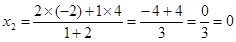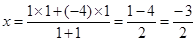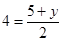NCERT Solutions Maths Class 10 Exercise 7.2

# NCERT Solutions Maths Class 10 Exercise 7.2

## Maths Class 10 Exercise 7.2

1. Find the coordinates of the point which divides the join of (–1, 7) and (4, –3) in the ratio 2 : 3.

Solution: Let x1 = –1, x2 = 4, y1 = 7 and y2 =  –3, m1 = 2 and m2 = 3

Let’s use the section formula to find the coordinates of a point which divides the join of (–1, 7) and (4, –3) in the ratio 2 : 3.

Therefore, the coordinates of the point are (1, 3) which divides the join of (–1, 7) and

(4, –3) in the ratio 2 : 3.

2. Find the coordinates of the points of trisection of the line segment joining (4, –1) and (–2, –3).

Solution:

We want to find the coordinates of the points of trisection of the line segment joining (4, –1) and (–2, –3).

We are given AC = CD = DB

We want to find the coordinates of point C and D.

Let the coordinates of the point C be (x1, y1) and the coordinates of the point D be (x2, y2).

Clearly, the point C divides the line segment AB in the ratio 1 : 2 and the point D divides the line segment AB in the ratio 2 : 1.

Let’s use the section formula to find the coordinates of point C which divides the join of A(4, –1) and B(–2, –3) in the ratio 1 : 2.

Let’s use the section formula to find the coordinates of point D which divides the join of A(4, –1) and B(–2, –3) in the ratio 2 : 1.Therefore, the coordinates of the point C are (2, –5/3 ) and the coordinates of the point D are (0, –7/3).

3. To conduct Sports Day activities, in your rectangular shaped school ground ABCD, lines have been drawn with chalk powder at a distance of 1 m each. 100 flower pots have been placed at a distance of 1 m from each other along AD, as shown in the figure. Niharika runs ¼ th of the distance AD on the 2nd line and posts a green flag. Preet runs 1/5 th of the distance AD on the eighth line and posts a red flag. What is the distance between both the flags? If Rashmi has to post a blue flag exactly halfway between the line segment joining the two flags, where should she post her flag?

Solution: Niharika runs ¼ th of the distance AD on the 2nd line and posts a green flag.

There are 100 flower pots between A and D. It means, she stops at 25th flower pot.

Therefore, the coordinates of the point where she stops are (2, 25).

Preet runs 1/5 th of the distance AD on the eighth line and posts a red flag. There are 100 flower pots between A and D. It means, she stops at 20th flower pot.

Therefore, the coordinates of the point where she stops are (8, 20).

Let’s use the distance formula to find the distance between points (2, 25) and (8, 20).

Rashmi posts a blue flag exactly halfway between the line segment joining the two flags.

Using section formula to find the coordinates of this point, we get

x = (2 + 8)/2 = 10/2 = 5

y = (25 + 20)/2 = 45/2

Therefore, the coordinates of the point, where Rashmi posts her flag are (5, 45/2).

It means that she posts her flag in 5th line after covering 45/2 = 22.5 m of distance.

4. Find the ratio in which the line segment joining the points (–3, 10) and (6, –8) is divided by (–1, 6).

Solution: Let (–1, 6) divides the line segment joining the points (–3, 10) and (6, –8) in the ratio k : 1.

k – 1 = 6k − 3

−7k = −2

k2/7

Therefore, the ratio is 2/7 : 1 which is equivalent to 2 : 7.

Therefore, (–1, 6) divides the line segment joining the points (–3, 10) and (6, –8) in the ratio 2 : 7.

5. Find the ratio in which the line segment joining A(1, –5) and B(–4, 5) is divided by the x-axis. Also find the coordinates of the point of division.

Solution: Let the coordinates of the point of division be (x, 0) and let it divides the line segment joining A(1, –5) and B(–4, 5) in the ratio k : 1.

Using the section formula, we get

Using equation (ii), we get

–5 + 5k = 0

5k = 5

k = 1

Putting the value of k in equation (i), we getTherefore, the point (–3/2, 0) on x-axis divides the line segment joining A(1, –5) and B(–4, 5) in 1 : 1.

6. If (1, 2), (4, y), (x, 6) and (3, 5) are the vertices of a parallelogram taken in order, find x and y.

Solution: Let A(1, 2), B(4, y), C(x, 6) and D(3, 5) are the vertices of the parallelogram ABCD.

We know that the diagonals of a parallelogram bisect each other. It means that the coordinates of the midpoint of diagonal AC would be the same as the coordinates of the midpoint of the diagonal BD. …… (i)

Using the section formula, the coordinates of the midpoint of AC are:Using the section formula, the coordinates of the midpoint of BD are:

1 + x = 7

x = 6

Again, according to condition (i), we also have8 = 5 + y

y = 3

Therefore, x = 6 and y = 3.

7. Find the coordinates of a point A, where AB is the diameter of a circle whose centre is (2, –3) and B is (1, 4).

Solution: We have to find the coordinates of the point A. We are given that AB is the diameter and the coordinates of the centre are (2, –3) and the coordinates of the point B are (1, 4).

Let the coordinates of the point A be (x, y).

4 = x + 1

x = 3

−6 = 4 + y

y = −10

Therefore, the coordinates of the point A are (3, –10).

8. If A and B are (–2, –2) and (2, –4), respectively, find the coordinates of P such that AP = 3/7 AB and P lies on the line segment AB.

Solution: The coordinates of points A and B are A(–2, –2) and B(2, –4).

It is given that AP = 3/7 AB

PB = AB – AP = AB – 3/7 AB = 4/7 AB

So, we have AP : PB = 3 : 4

Let the coordinates of the point P be (x, y).

Using the section formula to find the coordinates of point P, we getTherefore, the coordinates of the point P are (–2/7, –20/7).

9. Find the coordinates of the points which divide the line segment joining A(–2, 2) and B(2, 8) into four equal parts.

Solution: We have two points A(–2, 2) and B(2, 8).

Let P, Q and R be the points which divide the line segment AB into 4 equal parts.

Let the coordinates of the points P(x1, y1), Q (x2, y2) and R(x3, y3).

We know that AP = PQ = QR = RB.

It means that the point P divides the line segment AB in the ratio 1 : 3.

Using the section formula to find the coordinates of the point P, we get

Since, AP = PQ = QR = RB.

It means that the point Q is the midpoint of AB.

Using the section formula to find the coordinates of the point Q, we get

Since, AP = PQ = QR = RB.

It means that the point R divides the line segment AB in the ratio 3 : 1.

Using the section formula to find the coordinates of the point P, we get

Therefore, P(–1, 7/2), Q(0, 5) and R(1, 13/2) are the coordinates of the points which divide the line segment AB in four equal parts.

10. Find the area of a rhombus if its vertices are (3, 0), (4, 5), (–1, 4) and (–2, –1) taken in order. [Hint: Area of a rhombus = ½ (product of its diagonals)]

Solution: Let A(3, 0), B(4, 5), C(–1, 4) and D(–2, –1) are the vertices of the rhombus ABCD.

Using the distance formula to find the length of the diagonal AC, we get

Using the distance formula to find the length of the diagonal BD, we getSince, area of a rhombus = ½ (product of its diagonals)

= ½ × AC × BD

= ½ × 4√2 × 6√2

= 24 sq. units